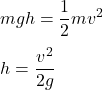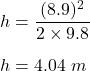Question

A 25 kg child is riding on a swing. If the child travels 8.9 m/s at the bottom of their swing, how high into the air is the child able to swing?

1.h = 4.04 m

Explanation:

Given that,

Mass of a child, m = 25 kg

The speed of the child at the bottom of the swing is 8.9 m/s

We need to find the height in the air is the child is able to swing. Let the height is h. Using the conservation of energy such that,Put all the values,So, the child is able to go at a height of 4.04 m.